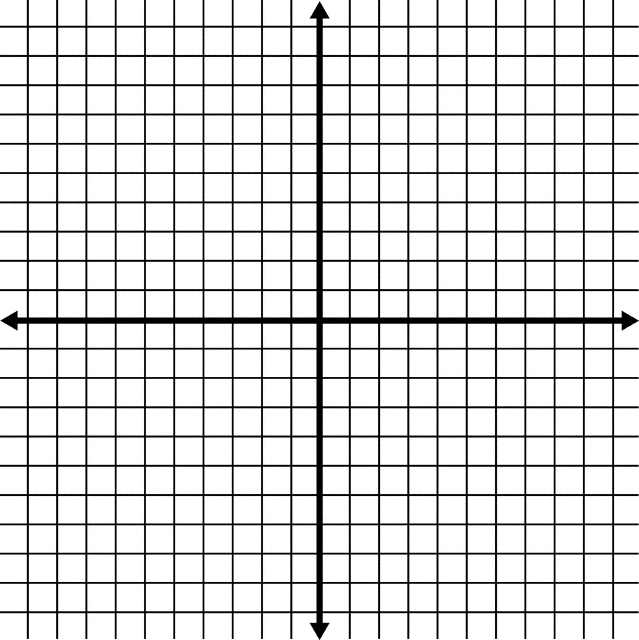# Cartesian Coordinate System

Introduction

good day!

The Cartesian coordinate system is a system that is used to create visual representations of equations. These representations are made on a grid called the Cartesian Plane.

The Cartesian Plane can be thought of as a map. A map allows us to find the location of something or someone by using street names and numbers. Just like a map, The Cartesian Plane allows us to find the location of a point.You may watch the story behind  Cartesian Plane and how mathematician  Rene Descartes discover the cartesian plane.

## X and Y AxisThe left-right (horizontal) direction is commonly called X.The up-down (vertical) direction is commonly called Y.
 Put them together on a graph ...## Writing Coordinates

The coordinates are always written in a certain order:

• the horizontal distance first,
• then the vertical distance.

This is called an "ordered pair" (a pair of numbers in a special order)

And usually the numbers are separated by a comma, and parentheses are put around the whole thing like this:

(3,2)

Lets do some of the exercise!

In a one whole sheet of paper answer the following.

I. Identify in which quadrant the following points

(-3, 12),(2,1),(-1,-1),(1,-1)

II. Find the distance between two points

1.(-3, 12),(2,1)

2.(-1,-1),(1,-1)

III. Label the Cartesian planeProcess

I.  These regions are called quadrants. They are numbered from 1 to 4, with Roman numerals, counter clockwise,  around a central point of origin.Until now, we only knew the origin O and the first quadrant. In it, the coordinates X and Y are always positive numbers.

• X positive means that the position is to the right of the origin.
• Y positive means that it is above the origin.

So, in this quadrant, (X,Y) are positive. We can write them abbreviated as (+,+).The yellow dot is on the coordinates (3,3). Three positions to the right of the origin and three above.

The first negative coordinate appears in this quadrant. The positive values tell us how many positions to count to the right or above the origin, X and Y respectively. In the same way, the negative values indicate how many positions we count to the left or below the origin of the X and Y axes. For example, if the X coordinate has a value of (-5) it means that it is 5 positions to the left of the origin. If the Y coordinate has the value of (-1), it means that it is one position below the origin.

• X negative indicates that the position is to the left of the origin.
• Y positive that it is above the origin.

Thus (X,Y) are written (-,+).In the example, the green dot is on the coordinates (-3,1). Three positions to the left of the origin and one position above.

Here, both values are negative.

• X negative indicates that the position is to the left of the origin.
• Y negative that it is below the origin.

Therefore (X,Y) are written (-,-).The red point is on the coordinates (-2,-5). Two positions to the left of the origin and five positions below.

The last quadrant is to the right and below the origin. The values of X and Y will be positive and negative respectively.

• X positive indicates that the position is to the right of the origin.
• Y negative that it is below the origin.

In other words (X,Y) are written (+,-).The blue dot is on the coordinates (4,-4). Four positions to the right of the origin and four below.

## II. Distance Between Two Points

We use the Pythagoras Theorem to derive a formula for finding the distance between two points in 2- and 3- dimensional space.

Let P = (x 1, y 1) and Q = (x 2, y 2) be two points on the Cartesian plane (see picture below).Then from the Pythagoras Theorem we find that the distance between P and Q isIn a similar way, it can be proved that if P = (x 1, y 1, z1) and Q = (x 2, y 2, z2) are two points in the 3-dimensional space, the distance between P and Q isIV.Evaluation

For test I,

• 2 points each correct plotting

For test II,

• 5 points each correct answer and solution
• 3 points if there are some missing part in the solution

For test III.

• 7 points for the complete labeling
• 5 points if 1-2 parts are missing
• 3 points if 3-below are missing

TOTAL ; 25 points

Conclusion

Cartesian plane is not only for mathematics but also use as map to know the location.

The Cartesian Plane

• is sometimes referred to as the x-y plane or the coordinate plane and is used to plot data pairs on a two-line graph.
• named after the mathematician Rene Descartes who originally came up with the concept.
• Cartesian planes are formed by two perpendicular number lines intersect.
Credits
Teacher Page

Soliven, Evangeline

Ed tech 2